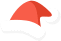WordPress как на ладоникласс не описан

# Text_Diff_Renderer{} WP 1.0

A class to render Diffs in different formats.

This class renders the diff in classic diff format. It is intended that this class be customized via inheritance, to obtain fancier outputs.

Copyright 2004-2010 The Horde Project (http://www.horde.org/)

Хуков нет.

Null. Ничего.

### Использование

```\$Text_Diff_Renderer = new Text_Diff_Renderer();
// use class methods```

### Заметки

• Пакет: Text_Diff

### Код Text_Diff_Renderer{} Text Diff Renderer{} WP 5.5.3

```class Text_Diff_Renderer {

/**
* Number of leading context "lines" to preserve.
*
* This should be left at zero for this class, but subclasses may want to
* set this to other values.
*/

/**
* Number of trailing context "lines" to preserve.
*
* This should be left at zero for this class, but subclasses may want to
* set this to other values.
*/
var \$_trailing_context_lines = 0;

/**
* Constructor.
*/
function __construct( \$params = array() )
{
foreach (\$params as \$param => \$value) {
\$v = '_' . \$param;
if (isset(\$this->\$v)) {
\$this->\$v = \$value;
}
}
}

/**
* PHP4 constructor.
*/
public function Text_Diff_Renderer( \$params = array() ) {
self::__construct( \$params );
}

/**
* Get any renderer parameters.
*
* @return array  All parameters of this renderer object.
*/
function getParams()
{
\$params = array();
foreach (get_object_vars(\$this) as \$k => \$v) {
if (\$k == '_') {
\$params[substr(\$k, 1)] = \$v;
}
}

return \$params;
}

/**
* Renders a diff.
*
* @param Text_Diff \$diff  A Text_Diff object.
*
* @return string  The formatted output.
*/
function render(\$diff)
{
\$xi = \$yi = 1;
\$block = false;
\$context = array();

\$ntrail = \$this->_trailing_context_lines;

\$output = \$this->_startDiff();

\$diffs = \$diff->getDiff();
foreach (\$diffs as \$i => \$edit) {
/* If these are unchanged (copied) lines, and we want to keep
* leading or trailing context lines, extract them from the copy
* block. */
if (is_a(\$edit, 'Text_Diff_Op_copy')) {
/* Do we have any diff blocks yet? */
if (is_array(\$block)) {
/* How many lines to keep as context from the copy
* block. */
\$keep = \$i == count(\$diffs) - 1 ? \$ntrail : \$nlead + \$ntrail;
if (count(\$edit->orig) <= \$keep) {
/* We have less lines in the block than we want for
* context => keep the whole block. */
\$block[] = \$edit;
} else {
if (\$ntrail) {
/* Create a new block with as many lines as we need
* for the trailing context. */
\$context = array_slice(\$edit->orig, 0, \$ntrail);
\$block[] = new Text_Diff_Op_copy(\$context);
}
/* @todo */
\$output .= \$this->_block(\$x0, \$ntrail + \$xi - \$x0,
\$y0, \$ntrail + \$yi - \$y0,
\$block);
\$block = false;
}
}
/* Keep the copy block as the context for the next block. */
\$context = \$edit->orig;
} else {
/* Don't we have any diff blocks yet? */
if (!is_array(\$block)) {
/* Extract context lines from the preceding copy block. */
\$context = array_slice(\$context, count(\$context) - \$nlead);
\$x0 = \$xi - count(\$context);
\$y0 = \$yi - count(\$context);
\$block = array();
if (\$context) {
\$block[] = new Text_Diff_Op_copy(\$context);
}
}
\$block[] = \$edit;
}

if (\$edit->orig) {
\$xi += count(\$edit->orig);
}
if (\$edit->final) {
\$yi += count(\$edit->final);
}
}

if (is_array(\$block)) {
\$output .= \$this->_block(\$x0, \$xi - \$x0,
\$y0, \$yi - \$y0,
\$block);
}

return \$output . \$this->_endDiff();
}

function _block(\$xbeg, \$xlen, \$ybeg, \$ylen, &\$edits)
{
\$output = \$this->_startBlock(\$this->_blockHeader(\$xbeg, \$xlen, \$ybeg, \$ylen));

foreach (\$edits as \$edit) {
switch (strtolower(get_class(\$edit))) {
case 'text_diff_op_copy':
\$output .= \$this->_context(\$edit->orig);
break;

break;

case 'text_diff_op_delete':
\$output .= \$this->_deleted(\$edit->orig);
break;

case 'text_diff_op_change':
\$output .= \$this->_changed(\$edit->orig, \$edit->final);
break;
}
}

return \$output . \$this->_endBlock();
}

function _startDiff()
{
return '';
}

function _endDiff()
{
return '';
}

{
if (\$xlen > 1) {
\$xbeg .= ',' . (\$xbeg + \$xlen - 1);
}
if (\$ylen > 1) {
\$ybeg .= ',' . (\$ybeg + \$ylen - 1);
}

// this matches the GNU Diff behaviour
if (\$xlen && !\$ylen) {
\$ybeg--;
} elseif (!\$xlen) {
\$xbeg--;
}

return \$xbeg . (\$xlen ? (\$ylen ? 'c' : 'd') : 'a') . \$ybeg;
}

{
}

function _endBlock()
{
return '';
}

function _lines(\$lines, \$prefix = ' ')
{
return \$prefix . implode("\n\$prefix", \$lines) . "\n";
}

function _context(\$lines)
{
return \$this->_lines(\$lines, '  ');
}

{
return \$this->_lines(\$lines, '> ');
}

function _deleted(\$lines)
{
return \$this->_lines(\$lines, '< ');
}

function _changed(\$orig, \$final)
{
return \$this->_deleted(\$orig) . "---\n" . \$this->_added(\$final);
}

}```